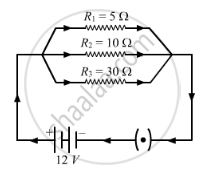Share

# In the Circuit Diagram Given Below, Three Resistors R1, R2, and R3 of 5 ω, 10 ω and 30 ω, Respectively Are Connected as Shown - CBSE Class 10 - Science

#### Question

In the circuit diagram  given below, three resistors R1, R2, and R3 of 5 Ω, 10 Ω and 30 Ω, respectively are connected as shownCalculate:
(a) current through each resistor.

(b) total current in the circuit.

(c) total resistance in the circuit.

#### Solution

(a) Let I1I2 and I3 be the current flowing through the resistors of 5 Ω, 100 Ω and 30 Ω, respectively.
According to Ohm's law, V = IR
Here, V = 12 V and R = 5 Ω
V/R
I1 = 12/5 = 2.4 A
I2 = 12/10 = 1.2 A
I3 = 12/30 = 0.4 A

(b) Total current through the circuit, I = I1 + I2 + I3
=  2.4 A + 1.2 A + 0.4 A
= 4 A

(c) The resistors of 5 Ω, 100 Ω and 30 Ω are connected in parallel. Therefore, the net resistance will be:
1/ R = 1/R1 + 1/R2 + 1/R3
1/R = (1/5) + (1/10) + (1/30)
1/R = (6 + 3 +1)/30
I/R = 10/30
R = 3 Ω

Is there an error in this question or solution?

#### Video TutorialsVIEW ALL 

Solution In the Circuit Diagram Given Below, Three Resistors R1, R2, and R3 of 5 ω, 10 ω and 30 ω, Respectively Are Connected as Shown Concept: Electric Current and Circuit.
S